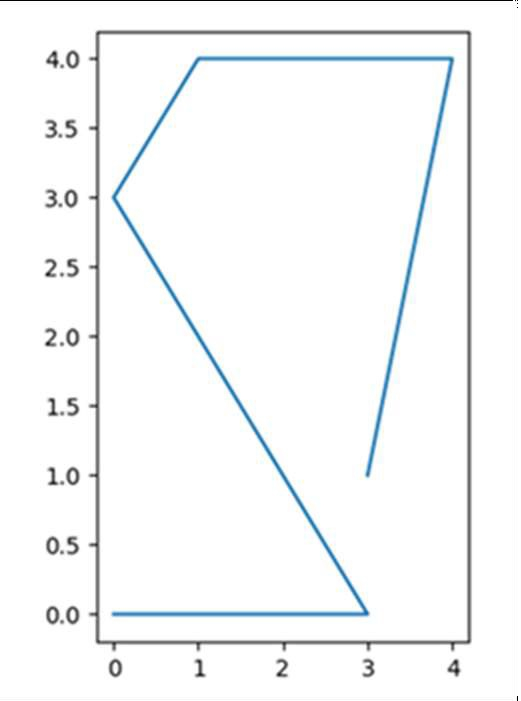# What is the difference between drawing plots using plot, axes or figure in matplotlib?

Let’s understand the difference between plot, axes, and figure with an example.

Plot − Plot helps to plot just one diagram with (x, y) coordinates.

Axes − Axes help to plot one or more diagrams in the same window and sets the location of the figure.

Figure − This method provides a top-level container for all the plot elements.

We can follow these steps to replicate the differences among them −

• Create a new figure, or activate an existing figure, using plt.figure().

• Add an axis to the figure as part of a subplot arrangement, using plt.add_subplot(xyz) where x is nrows, y is ncols and z is the index. Here taking x = 1(rows), y = 2(columns) and z = 1(position).

• Draw the line bypassing the lists into the plt.plot() method.

• To show the diagram, use the plt.show() method.

## Example

We will use plot, axes, and figure in the following code −

import matplotlib.pyplot as plt

fig = plt.figure()             # Activating figure

ax1 = fig.add_subplot(121)     # Setting up figure position

plt.plot([3, 4, 1, 0, 3, 0], [1, 4, 4, 3, 0, 0]) # Drawing lines in the
activated figure.

plt.show()

## Output